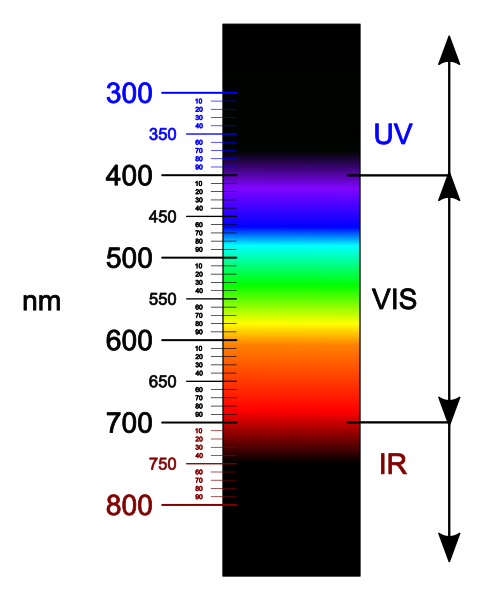Courses
Courses for Kids
Free study material
Free LIVE classes
More# The number of wavelength in the visible spectrum is:A) $4000$B) $6000$C) $2000$D) infiniteVerified
289.2k+ views
Hint: In general, there are seven types of the electromagnetic radiations. In these seven types of the electromagnetic radiations, the visible light comes in fourth place. And the visible light has different colours and different wavelengths.

Complete step by step solution:
Electromagnetic waves exist with a large amount of the range of frequencies. This continuous and large amount of frequencies is commonly known as the electromagnetic spectrum. This long range of the spectrum is divided into different types of waves. In electromagnetic waves, there are seven types, they are gamma rays, x-rays, UV rays, visible light, infrared waves, microwaves radiation and radio waves. And these seven types of electromagnetic waves have different wavelengths and different frequencies.

Electromagnetic radiation or waves with a wavelength between $400\,nm$ to $700\,nm$ are called the visible light spectrum. These wavelengths are detected by the human eyes. So, this spectrum is called the visible light spectrum. The wavelengths which are larger than the $700\,nm$ are called infrared waves. And the wavelengths which are smaller than the $400\,nm$ are called ultraviolet waves. Sometimes, these two rays are also called the lights. White light is the combination of the lights of different wavelengths in the visible spectrum. Passing the white light through the prism, it splits up into some colours of light with the wavelength range of the $400\,nm$ and $700\,nm$.The colour of the visible light is VIBGYOR which means violet, indigo, blue, green, yellow, orange and red. The minimum wavelength is for the violet $400\,nm$ and the maximum wavelength is for the red light $700\,nm$.

Thus, the visible spectrum has an infinite number of wavelengths.

Hence, the option (D) is the correct answer.

Note: The light that excites the human visual system is a very small portion of the electromagnetic spectrum. A rainbow shows the optical (visible) part of the electromagnetic spectrum; infrared (if it could be seen) would be located just beyond the red side of the rainbow with ultraviolet appearing just beyond the violet end.
Last updated date: 28th May 2023
Total views: 289.2k
Views today: 3.46k